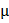# A sample of radioactive material A, that has an activity of 10m Ci(l Ci=3.71010decays/s), has twice the number of nuclei as another sample of a different radioactive maternal B which has an activity of 20mCi.  The correct choice for hall-lives of A and B an would then be respectively: a. 20 days and 5 days b. 20 days and 10 days c. 5 days and 10 days d. 10days and 40days

## Question ID - 50055 :- A sample of radioactive material A, that has an activity of 10m Ci(l Ci=3.71010decays/s), has twice the number of nuclei as another sample of a different radioactive maternal B which has an activity of 20mCi.  The correct choice for hall-lives of A and B an would then be respectively: a. 20 days and 5 days b. 20 days and 10 days c. 5 days and 10 days d. 10days and 40days

3537

Activity A=N

For A    10=(2N0)A

For B     20=N0BB=4A(T1/2)A=4(T1/2)B

Next Question :

Consider a tank made of glass (reiractive index 1.5) with a thick bottom.  It is filled with a liquid of refractive index., A student finds that, irrespective of what the incident angle i(see figure0 is for a beam of light entering the liquid, is never reflected from the liquid glass interface happen, the minimum value ofis:a.b.c.d.